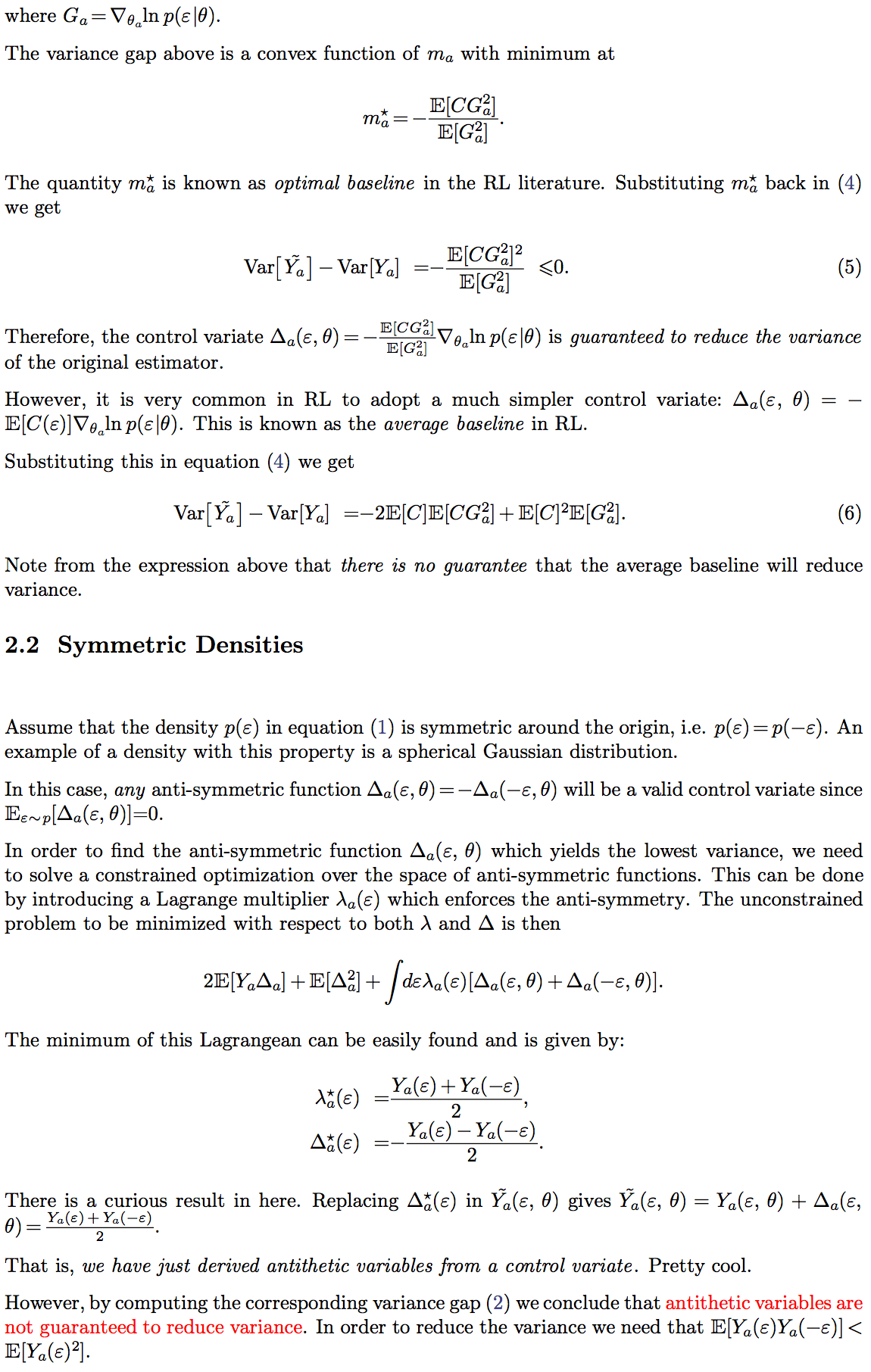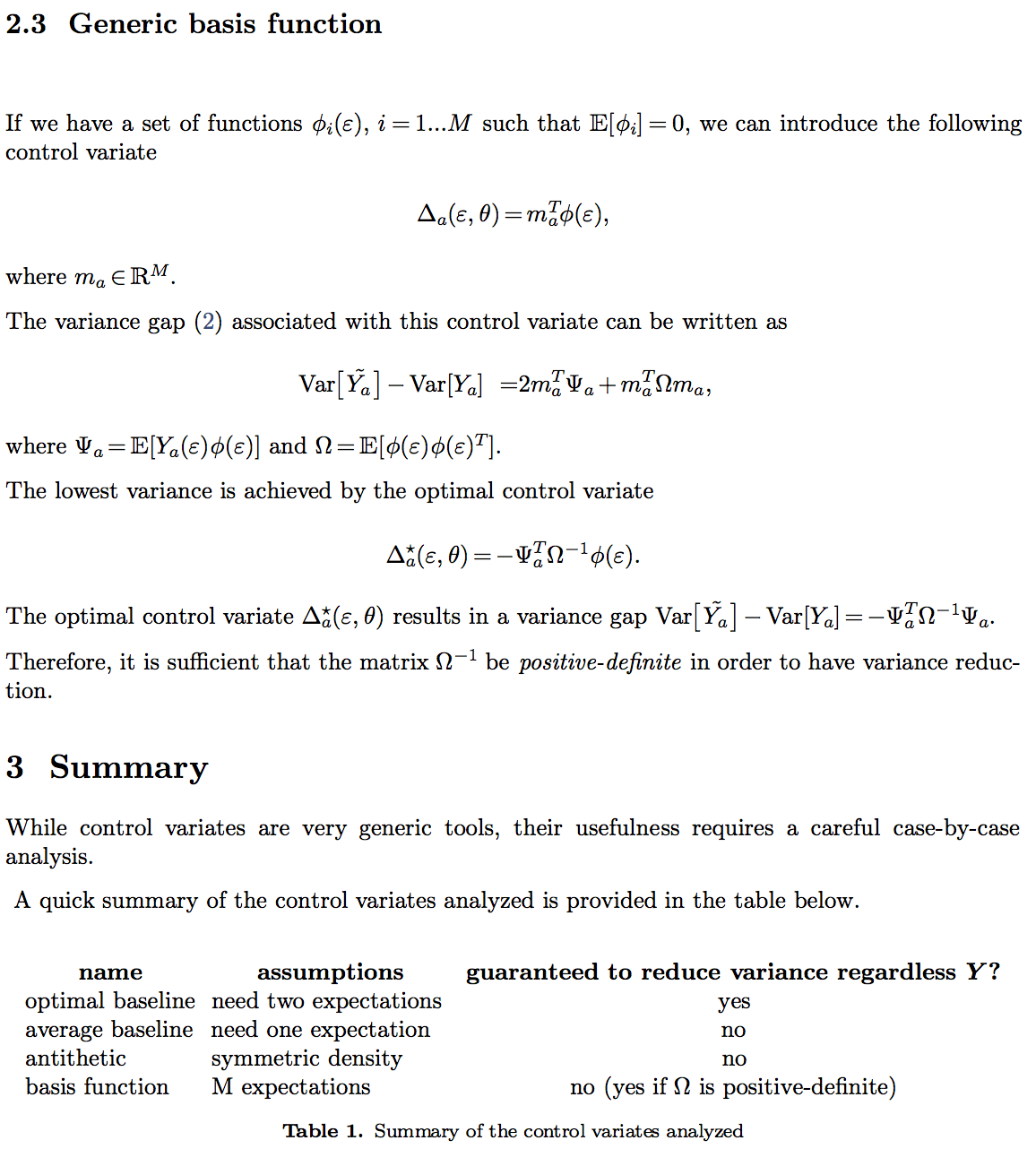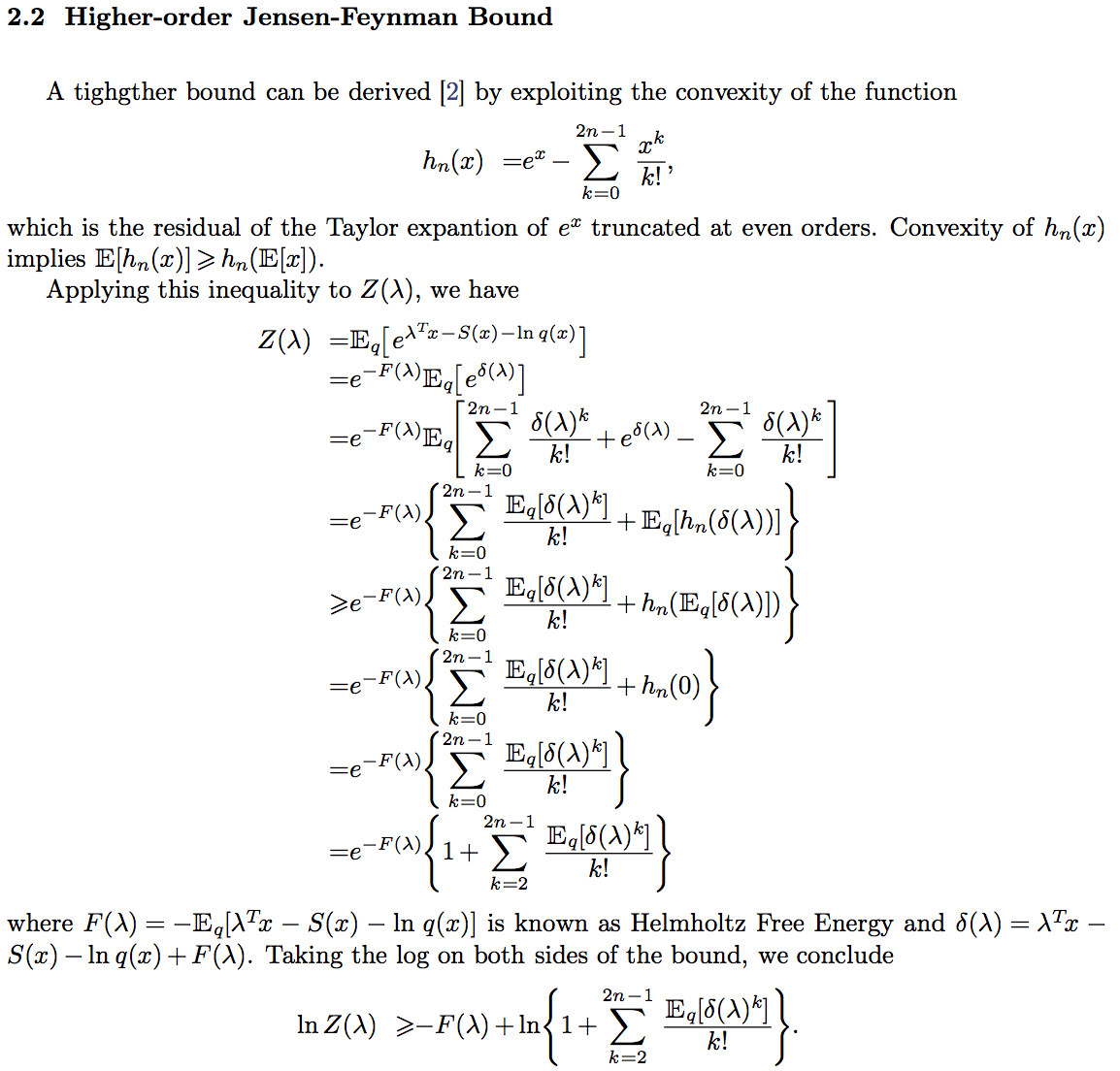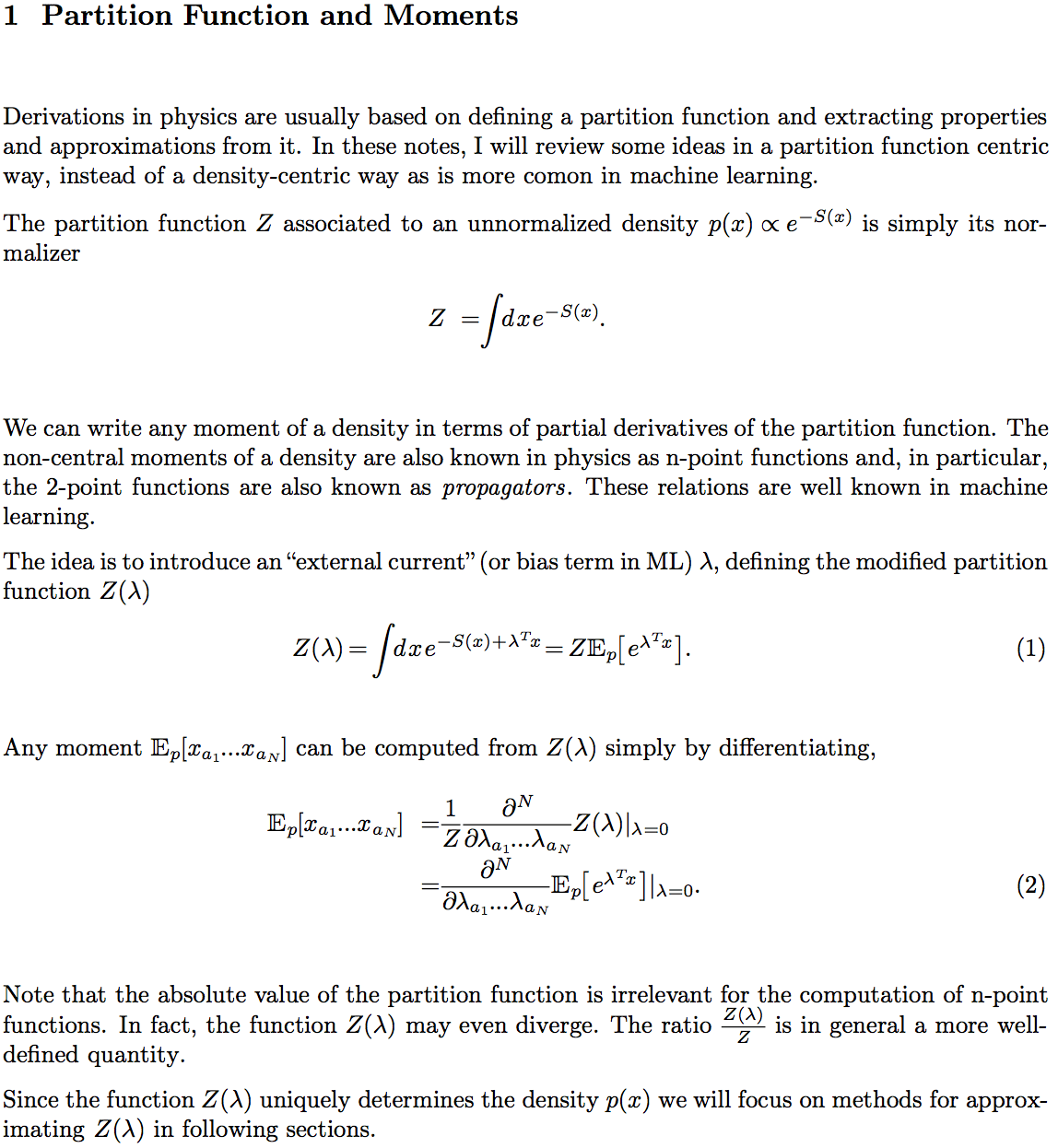# Approximating Free Energies

Merry Christmas all!

By now, I hope all machine learners are convinced of the importance of variational methods for approximated inference and learning in general. Specially given the fast increase in popularity of those methods (NIPS15, NIPS14).

As a follow up of my posts on partition functions ( part1, part2 and part3 ), I was inspired by a couple of papers this last NIPS ( paper1, paper2 ) to expand/review a little more the methods for approximating partition functions and free energies in statistical mechanics.The full pdf of this post can be found here.

# Motivation

For many problems in machine learning (ranging from Generative Models to Reinforcement Learning), we rely on Monte Carlo estimators of gradients for optimization. Often, the noise in our gradient estimators is a major nuisance factor affecting how close we can get to local optima.

There are many tricks around the corner to improve on this issue. A popular one is the “bias removal trick” widely known in the Reinforcement Learning literature.

Many of these tricks are particular cases of what is known as a control variate ( link1, link2, link3 ) a very generic method for variance reduction.

In this post I will try to characterize a few interesting and potentially useful applications of control variates and discuss their limitations.If you happen to know more interesting facts, theorems or use cases of control variates please let me know.

The pdf source of this post can be found here.

# Partition Functions and Higher-order Jensen Inequalities (3/3)

When trying to compute variational bounds (as derived in the previous post), a naive attempt to approximate the involved expectations (e.g. using a Taylor expansion) may destroy the bound.

This is where the Higher-order Jensen-Feynman inequality comes in. It allows us to do a higher-order polynomial expansion without destroying the variational bound.# Partition Functions and the Jensen-Feynman Inequalities (2/3)## Partition Functions and Moments (1/3)

### Image# Useful Inequalities for Variational Inference

Variational Inference is a technique which consists in bounding the log-likelihood ln p(x) defined by a model with latent variables p(x,z)=p(x|z)p(z) through the introduction of  a variational distribution q(z|x) with same support as p(z):Often the expectations in the bound F(x) (aka, ELBO or Free Energy) cannot be solved analytically.

In some cases, we can make use of a few handful inequalities which I quickly summarize below.

Some of these inequalities introduce new variational parameters. Those should be optimized jointly with all the other parameters to minimize the ELBO.